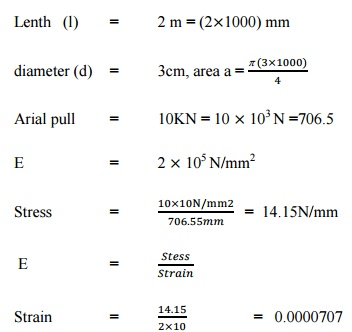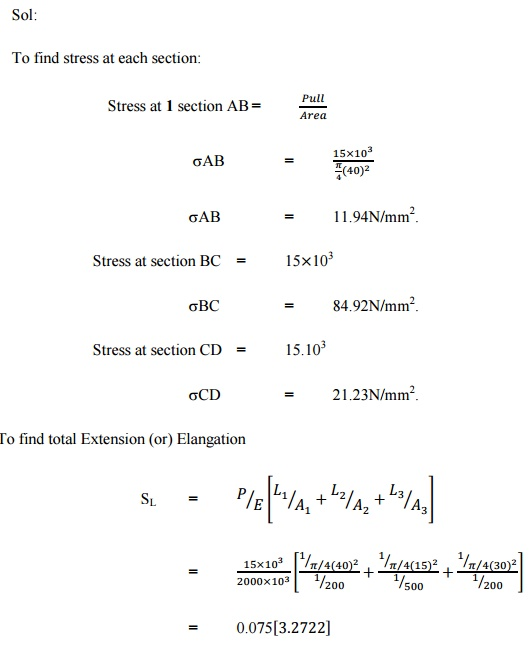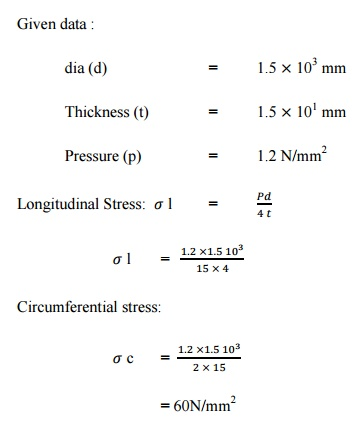Home | | Strength of Materials for Mechanical Engineers | Solved Answers: Stress, Strain and Deformation of Solids

# Solved Answers: Stress, Strain and Deformation of Solids

Mechanical - Strength of Materials - Stress, Strain and Deformation of Solids

Problem - 1:

A mild steel rod 2m long &3cm diameter is subjected to an axial pull of 10kN. E for Youngs modulus for steel is 2 x 105 N/mm2. Find the stress, strain.

Sol:Problem - 2:

A steel bar 900mm long its 2ends are 40mm &30mm in diameters &the length of each rod is 200mm. The middle portion of the bar is 15mm in diameters &500mm in long if the bar is subjected an axial tensile load of 15KN. Determine the stress in each section &total extension. Take E=200 103N/mm2.

Sol:Problem –3:

The following are the results of a tensile on test on a molested rod is 16 mm gaugh length is 50 mm, load at proportionality limit is 48.5 kN. Extension at the proportionally limit is 0.05mm. Load at yield point is 50.3 KN ultimate load is 90KN. Final length between gauge points is 64mm, dia of the neck at   fracture   is   13.7   mm.   Determine   the e you Stress, % of elangation & % of reduction in area.

Given data:

Dia of rod d          =       16mm

Dia of Fracture of rod fd         =       13.7 mm

Gauge length l      =       50mm

Final length  \$  L  =       645 mm

Load at yield point                  =       50.3KN

Load at   limit       =       48.5 KN

Extension of ( inf ) limit \$   l   =       0.05.mmProblem - 4:

A Cylindrical pipe of diameter 1.5mm & thickness 1.5cm is subjected to an internal fluid pressure of 1.2 N/mm2. Find the longitudinal stress & hoop stress developed in the pipe.Problem - 5:

A Cyliner of internal dia 0.5m contains air at apressure of 7 N/mm2.If the maximum permissible stress induced in material is 80 N/mm2. Find the thickness of the cylinder .Problem - 6:

Calculate change in length, change in diameters, change in volume of the thin cylinder 100cm dia & 1cm thickness, 5m long E= 2 105 N/mm2 poisson’s ratio=0.3& internal(1/m)fluid (or pressure 3 N/mm2.Problem - 7:

A bar of 20mm diameter is tested in tension it is observed that when a lead of 40KN is applied the extension measured over a gauge length of 200mm us 0.12mm&contraction in diameter is 0.0036mm. Find    poisson’s    ratio,    young’s modulas.Study Material, Lecturing Notes, Assignment, Reference, Wiki description explanation, brief detail
Mechanical : Strength of Materials : Stress, Strain and Deformation of Solids : Solved Answers: Stress, Strain and Deformation of Solids |